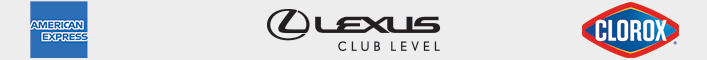/

# June 2021

SUNDAY MONDAY TUESDAY WEDNESDAY THURSDAY FRIDAY SATURDAY
30
+
31
+
01
+
02
+
03
+
04
+
05
+
06
+
07
+
08
+
09
+
10
+
11
+
12
+
13
+
14
+
15
+
16
+
17
+
18
+
19
+
20
+
21
+
22
+
23
+
24
+
25
+
26
+
27
+
28
+
29
+
30
+
01
+
02
+
03
+close (X)